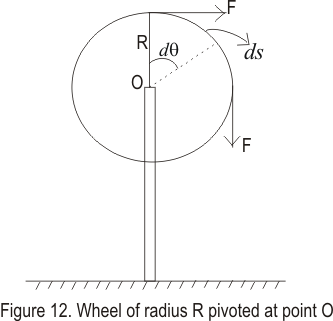# Work done and power in rotational motion

## work done and power in rotational motion

• We know that when we apply force on any object in direction of the displacement of the object ,work is said to be done
• Similarly force applied to the rotational body does work on it and this work done can be expressed in terms of moment of force (torque) and angular displacement θ
• Consider the figure given below where a force F acts on the wheel of radius R pivoted at point O .so that it can rotate through point O• This force F rotates the wheel through an angle dθ and dθ is small enough so that we can regard force to be constant during corresponding time interval dt
• Work done by this force is
dW=Fds
but ds=Rdθ
So
dW=FRdθ
• Now FR is the torque Τ due to force F.so we have
dW=Τdθ ----(19)
• if the torque is constant while angle changes from θ1 to θ2 then
W=Τ(θ21)=ΤΔθ ---(20)
Thus work done by the constant torque equals the product of the torque and angular displacement
• we know that rate of doing work is the power input of torque so
P=dW/dt=Τ(dθ/dt)=Τω
• In vector notation
P=Τ.ω